#### You may also like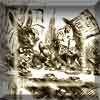### A Problem of Time

Consider a watch face which has identical hands and identical marks for the hours. It is opposite to a mirror. When is the time as read direct and in the mirror exactly the same between 6 and 7?The points P, Q, R and S are the midpoints of the edges of a non-convex quadrilateral.What do you notice about the quadrilateral PQRS and its area?

# Same Length

##### Age 11 to 16Challenge Level
The square with two equilateral triangles
Pablo from King's College Alicante used diagrams to form a clever proof: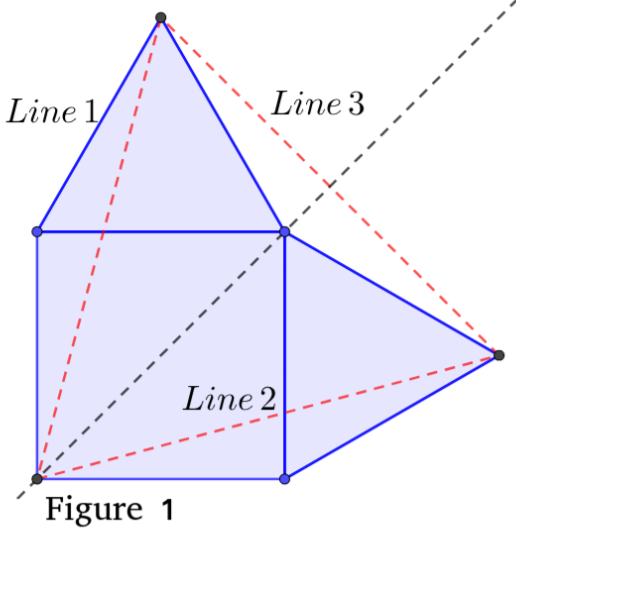One way to prove that the triangle created is equilateral is to show that each side is the same.
If the sides of the triangle are named 1, 2 and 3, then it is clear (from Figure 1) that 1 and 2 are equal, by symmetry. It is a line of symmetry because the quadrilateral is a square and thus the equilateral triangles formed are identical. The square has a line of symmetry as its diagonal.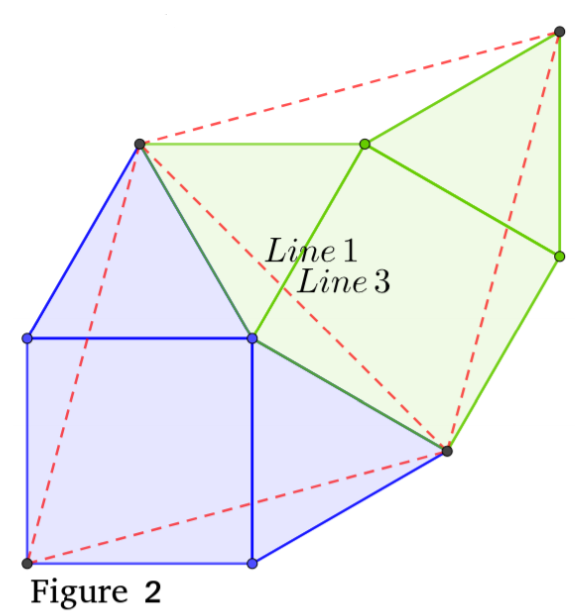Secondly, a copy of the diagram can be placed next to the square and triangles, as seen in (Figure 2). The shapes fit perfectly because the angles around the top right corner of the square add up to 360 º:
90 + 90 + 60 + 60 + 60 = 360 º

Line 3 is now on Line 1 of the copy. Therefore Line 3 and Line 1 are equal.

Since Line 1 = Line 2 and Line 1 = Line 3,
Line 1 = Line 2 = Line 3
Therefore it is an equilateral triangle.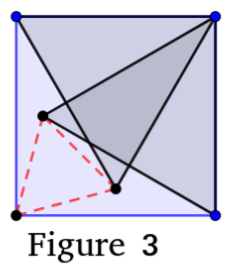If the equilateral triangles are drawn on the inside, another triangle is formed. A diagram can be seen in (Figure 3).

Again, by symmetry Line 1 and 2 are equal.

In (Figure 4) a copy of the diagram has been placed on the side of the
rightmost equilateral triangle.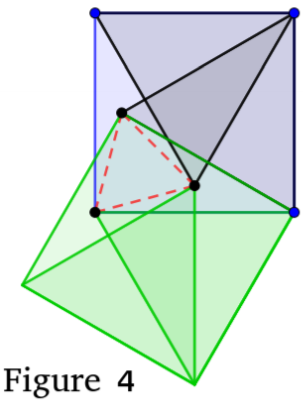Since the length of the side of that equilateral triangle is the same as the side length of the square (by definition) the copy fits perfectly on that side. The copy of the red triangle is exactly on top of the original.

The only thing that has changed is which side is which. The kite that contains two sides of the red triangle has been rotated. This shows that the 3rd side is equal to the first.

Line 1 = Line 2 = Line 3
Therefore it is an equilateral triangle.

Kian from King Edward VII School in the UK used angles to prove that the triangle formed is equilateral: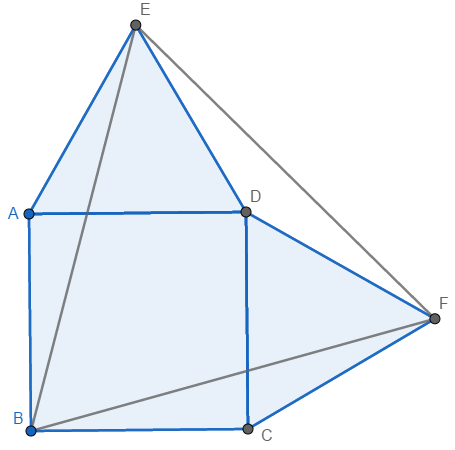Let the vertices of the square be $A, B, C, D,$ and let $E$ and $F$ be the points outside the square such that $AED$ and $DFC$ are equilateral triangles, as shown in the diagram.

Firstly, note that $AB = AD$, as they are sides of a square, and $AD = AE,$ as they are sides of an equilateral triangle, which means that $AB = AD = AE.$ Importantly, $AB = AE.$ This tells us that triangle $ABE$ is isosceles, with $A\hat{B}E = A\hat EB.$

$B\hat AE = B\hat AD + D\hat AE = 90^\circ + 60^\circ = 150^\circ,$ so $A\hat EB = A\hat BE = (180^\circ-150^\circ)\div2=15^\circ$

Now, $A\hat BC = 90^\circ$ as it is an interior angle of a square, so $E\hat BF = 90^\circ - A\hat BE - C\hat BF = 60^\circ.$

Additionally, $AB = BC, AE = CF$ and $B\hat AE = B\hat CF = 150^\circ,$ which means that triangles $ABE$ and $CBF$ are congruent under SAS, and $BE = BF.$ Hence, triangle $BEF$ is isosceles, with $B\hat EF = E\hat FB = (180^\circ-60^\circ)\div2 = 60^\circ.$ All three angles of triangle $BEF$ are equal to $60^\circ$, so it is equilateral.

Jake from GSAL in the UK used the cosine rule: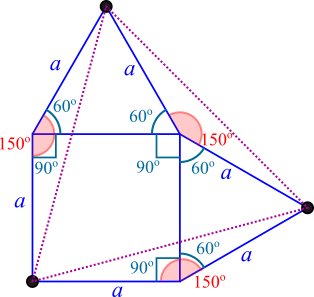[Notice] the isosceles triangles forming around the equilateral triangle's lines - both sides must be '$a$' since one side of the equilateral triangles is equal to the square's side.

[These] can be used in the cosine rule, $c^2=a^2+b^2-2ab\cos x$, where $a$ and $b$ are both '$a$'. In all circumstances $x$ can be found to be 150 so it works out into the same result of all sides being equal (in the new triangle) to $\sqrt{2a^2-2a^2\cos{150}}$.

Notice that Jake didn't actually need to apply the cosine rule - the three isosceles triangles must be congruent, since they have the same shorter sides and angle. So their longer sides must also all be equal.

The two equilateral triangles
Pablo and Kian both used similar triangles. This is Pablo's work: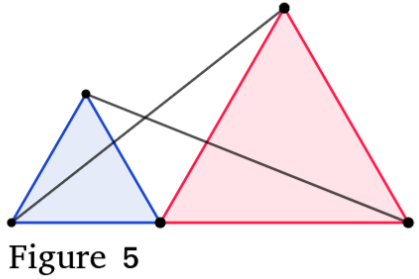(Figure 5) shows the diagram. The segments from the tips of the equilateral triangles to the opposite end of the baseline are equal.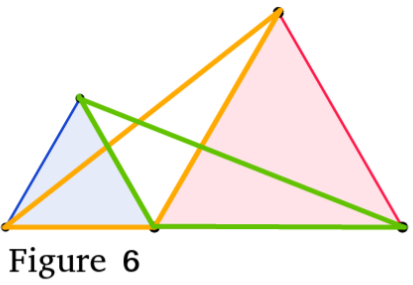This can be proven using congruent triangles. The two triangles in question are shown in (Figure 6). The biggest angle for both of them is 120 º (180 º for a straight line minus 60 º from the equilateral triangle). They share two sides, from the two equilateral triangles.

Therefore the triangles are congruent and so the last side for each is equal.

This problem can also be solved using Pythagoras' Theorem, as shown below.
Let the side length of the large equilateral triangle be $a$, and the side length of the small equilateral triangle be $b$.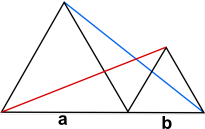Take the large equilateral triangle: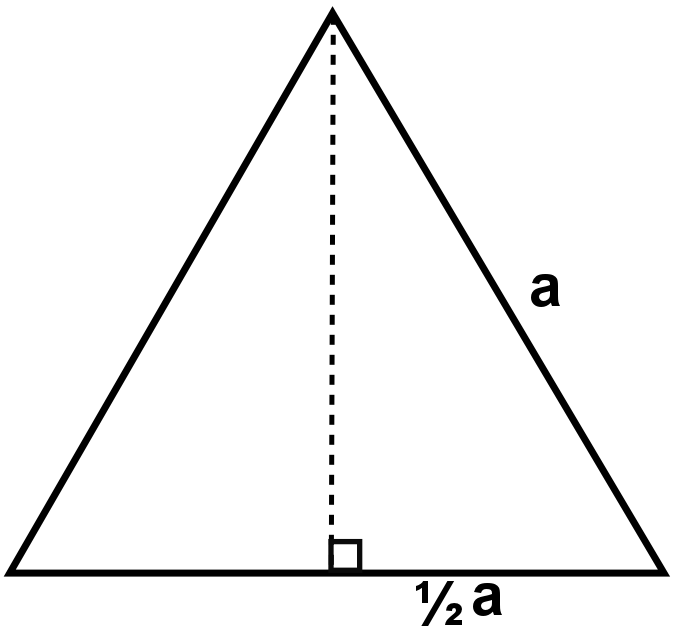Using Pythagoras, we find that the perpendicular height of the larger triangle is
$$\sqrt{a^2 - (\frac{1}{2} a)^2} = \frac{\sqrt{3}}{2}a.$$
Now take the triangle with the blue line as the hypotenuse: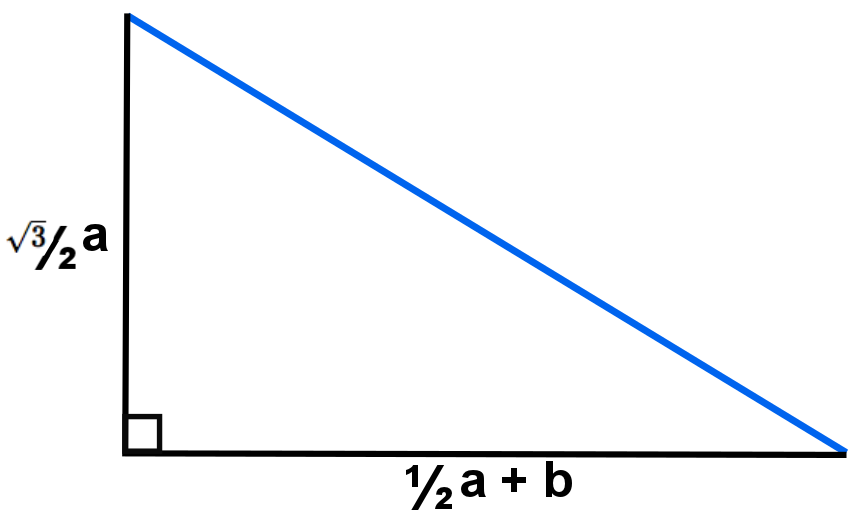By Pythagoras again, we find that the length of the blue line is given by
$$\textrm{blue line} = \sqrt{\frac{3}{4}a^2 + (\frac{1}{2}a+b)^2} = \sqrt{a^2 + ab + b^2}.$$

Similarly, the perpendicular height of the smaller equilateral triangle is
$$\sqrt{b^2 - (\frac{1}{2} b)^2} = \frac{\sqrt{3}}{2}b.$$
And so we can take the triangle with the red line as the hypotenuse: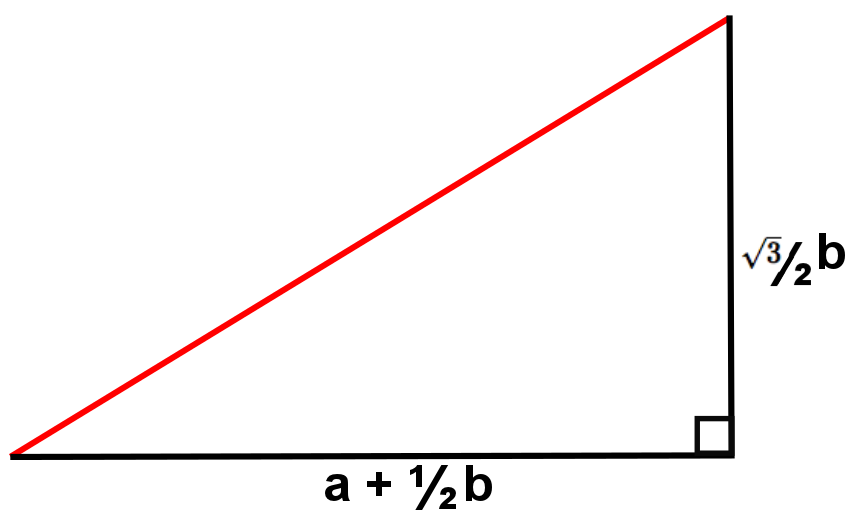to give that the length of the red line is
$$\textrm{red line} = \sqrt{(a+\frac{1}{2}b)^2 + \frac{3}{4}b^2} = \sqrt{a^2 + ab + b^2}.$$

So the red and blue lines are the same length.